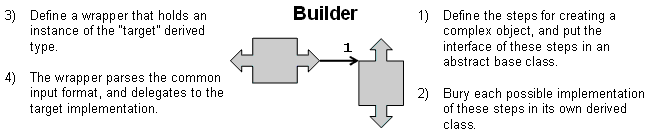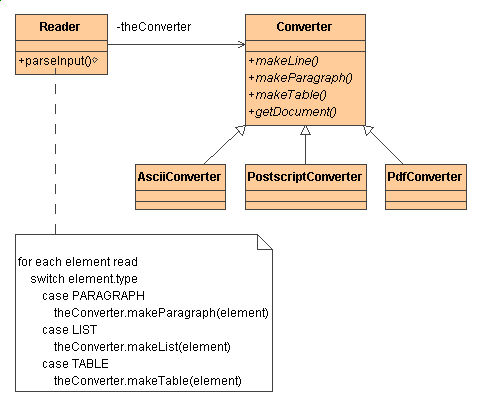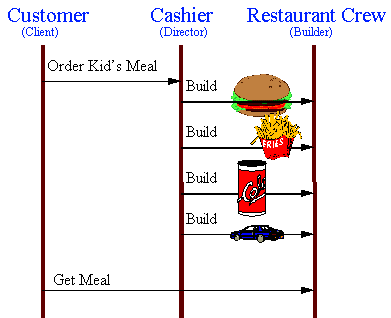# Builder

### Intent

• Separate the construction of a complex object from its representation so that the same construction process can create different representations. [GoF, p97]
• Parse a complex representation, create one of several targets.

### Problem

An application needs to create the elements of a complex aggregate. The specification for the aggregate exists on secondary storage and one of many representations needs to be built in primary storage.

### Structure SummaryStructure category:
wrapper + inheritance hierarchy
Similar patterns:   Bridge   Observer   State

### Discussion

Separate the algorithm for interpreting (i.e. reading and parsing) a stored persistence mechanism (e.g. RTF files) from the algorithm for building and representing one of many target products (e.g. ASCII, TeX, text widget). The focus/distinction is on creating complex aggregates.

The "director" invokes "builder" services as it interprets the external format. The "builder" creates part of the complex object each time it is called and maintains all intermediate state. When the product is finished, the client retrieves the result from the "builder".

Affords finer control over the construction process. Unlike creational patterns that construct products in one shot, the Builder pattern constructs the product step by step under the control of the "director".

### StructureThe Reader encapsulates the parsing of the common input. The Builder hierarchy makes possible the polymorphic creation of many peculiar representations or targets.### Example

The Builder pattern separates the construction of a complex object from its representation so that the same construction process can create different representations. This pattern is used by fast food restaurants to construct children's meals. Children's meals typically consist of a main item, a side item, a drink, and a toy (e.g., a hamburger, fries, Coke, and toy car). Note that there can be variation in the content of the children's meal, but the construction process is the same. Whether a customer orders a hamburger, cheeseburger, or chicken, the process is the same. The employee at the counter directs the crew to assemble a main item, side item, and toy. These items are then placed in a bag. The drink is placed in a cup and remains outside of the bag. This same process is used at competing restaurants. [Michael Duell, "Non-software examples of software design patterns", Object Magazine, Jul 97, p54]### Check list

1. Decide if a common input and many possible representations (or outputs) is the problem at hand.
2. Encapsulate the parsing of the common input in a Reader class.
3. Design a standard protocol for creating all possible output representations. Capture the steps of this protocol in a Builder interface.
4. Define a Builder derived class for each target representation.
5. The client creates a Reader object and a Builder object, and registers the latter with the former.
7. The client asks the Builder to return the result.

### Before and after

BeforeAfter
```// BEFORE - This implementation is arguably preferable.  Each
// table class encapsulates a different layout.

class JTable_Table {
private JTable m_table;

public JTable_Table( String[][] matrix ) {
m_table = new JTable( matrix.length, matrix.length );
TableModel model = m_table.getModel();
for (int i=0; i < matrix.length; ++i)
for (int j=0; j < matrix[i].length; ++j)
model.setValueAt( matrix[i][j], j, i );
}
public Component get_table() { return m_table; }
}

class GridLayout_Table {
private JPanel m_table = new JPanel();

public GridLayout_Table( String[][] matrix ) {
m_table.setLayout( new GridLayout(
matrix.length, matrix.length ) );
m_table.setBackground( Color.white );
for (int i=0; i < matrix[i].length; ++i)
for (int j=0; j < matrix.length; ++j)
m_table.add( new Label( matrix[j][i] ) );
}
public Component get_table() { return m_table; }
}

class GridBagLayout_Table {
private JPanel m_table = new JPanel();

public GridBagLayout_Table( String[][] matrix ) {
GridBagConstraints c = new GridBagConstraints();
m_table.setLayout( new GridBagLayout() );
m_table.setBackground( Color.white );
for (int i=0; i < matrix.length; ++i)
for (int j=0; j < matrix[i].length; ++j) {
c.gridx = i;
c.gridy = j;
m_table.add( new Label( matrix[i][j] ), c );
}     }
public Component get_table() { return m_table; }
}

public class BuilderDemo {
public static String[][] read_data_file( String file_name ) {
String[][] matrix = null;
try {
String line, cell = "";
String[] tokens;
boolean first_line = true;
int row=0, col=0;
while ((line = br.readLine()) != null) {
// Use "whitespace" to tokenize each line
// java.sun.com/docs/books/tutorial/extra/
//    regex/pre_char_classes.html
tokens = line.split( "\\s" );
int i = 0;
if (first_line) {
matrix = new String[Integer.parseInt( tokens )]
[Integer.parseInt( tokens )];
i = 2;
first_line = false;
}
for ( ; i < tokens.length; ++i)
if (tokens[i].equals( "" )) {
matrix[col][row++] = cell;
cell = "";
col = 0;
} else if (tokens[i].equals( "" )) {
matrix[col++][row] = cell;
cell = "";
} else {
cell += " " + tokens[i];
}
}
matrix[col][row] = cell;
br.close();
} catch( Exception ex ) { ex.printStackTrace(); }
return matrix;
}
public static void main( String[] args ) {
JFrame frame = new JFrame( "BuilderDemo - " + args );
frame.setDefaultCloseOperation( JFrame.EXIT_ON_CLOSE );
String[][] matrix = read_data_file( "BuilderDemo.dat" );
if (args.equals( "JTable_Table" ))
new JTable_Table( matrix ).get_table() );
else if (args.equals( "GridLayout_Table" ))
new GridLayout_Table( matrix ).get_table() );
else if (args.equals( "GridBagLayout_Table" ))
new GridBagLayout_Table( matrix ).get_table() );
frame.pack();
frame.setVisible( true );
}  }
```

```// AFTER - The main() creates a reader/parser, and configures
// it with a builder (an object that implements a standard
// interface and knows how to create one of many possible
// and delegates the construction to the configured builder.

// This implementation demonstrates the spirit of the Builder
// pattern -- but -- it is more intricate, and probably cannot
// be justified for this fairly limited context.

private Builder m_builder;

public Reader( Builder b ) { m_builder = b; }

public void construct( String file_name ) {
try {
String line, cell = "";
String[] tokens;
boolean first_line = true;
while ((line = br.readLine()) != null) {
tokens = line.split( "\\s" );
int i = 0;
if (first_line) {
m_builder.set_width_and_height(
Integer.parseInt( tokens ),
Integer.parseInt( tokens ) );
i = 2;
first_line = false;
}
for ( ; i < tokens.length; ++i)
if (tokens[i].equals( "" )) {
m_builder.build_cell( cell );
cell = "";
m_builder.start_row();
} else if (tokens[i].equals( "" )) {
m_builder.build_cell( cell );
cell = "";
} else {
cell += " " + tokens[i];
}
}
m_builder.build_cell( cell );
br.close();
} catch( Exception ex ) { ex.printStackTrace(); }
}  }

interface Builder {
void set_width_and_height( int width, int height );
void start_row();
void build_cell( String value );
Component get_result();
}

class JTable_Builder implements Builder {
private JTable     m_table;
private TableModel m_model;
private int i = 0, j = 0;

public void set_width_and_height( int width, int height ) {
m_table = new JTable( height, width );
m_model = m_table.getModel();
}
public void start_row() {
++i;
j = 0;
}
public void build_cell( String value ) {
m_model.setValueAt( value, i, j++ );
}
public Component get_result() { return m_table; }
}

class GridLayout_Builder implements Builder {
private JPanel m_panel = new JPanel();

public void set_width_and_height( int width, int height ) {
m_panel.setLayout( new GridLayout( height, width ) );
m_panel.setBackground( Color.white );
}
public void start_row() { }
public void build_cell( String value ) {
m_panel.add( new Label( value ) );
}
public Component get_result() { return m_panel; }
}

class GridBagLayout_Builder implements Builder {
private JPanel m_panel = new JPanel();
private GridBagConstraints c = new GridBagConstraints();
private int i = 0, j = 0;

public void set_width_and_height( int width, int height ) {
m_panel.setLayout( new GridBagLayout() );
m_panel.setBackground( Color.white );
}
public void start_row() {
++i;
j = 0;
}
public void build_cell( String value ) {
c.gridx = j++;
c.gridy = i;
m_panel.add( new Label( value ), c );
}
public Component get_result() { return m_panel; }
}

public class BuilderDemo {
public static void main( String[] args ) {
Builder target = null;
try {
target = (Builder) Class.forName(
args ).newInstance();
} catch( Exception ex ) { ex.printStackTrace(); }
parser.construct( "BuilderDemo.dat" );

JFrame frame = new JFrame( "BuilderDemo - " + args );
frame.setDefaultCloseOperation( JFrame.EXIT_ON_CLOSE );
frame.pack();
frame.setVisible( true );
}  }
```

### Rules of thumb

Sometimes creational patterns are complementory: Builder can use one of the other patterns to implement which components get built. Abstract Factory, Builder, and Prototype can use Singleton in their implementations. [GoF, p81, 134]

Builder focuses on constructing a complex object step by step. Abstract Factory emphasizes a family of product objects (either simple or complex). Builder returns the product as a final step, but as far as the Abstract Factory is concerned, the product gets returned immediately. [GoF, p105]

Builder often builds a Composite. [GoF, p106]

Often, designs start out using Factory Method (less complicated, more customizable, subclasses proliferate) and evolve toward Abstract Factory, Prototype, or Builder (more flexible, more complex) as the designer discovers where more flexibility is needed. [GoF, p136]